telescopeѲptics.net          ▪▪▪▪                                             CONTENTS

# 4.6. IMAGE Field curvature

Ideally, every point imaged by a telescope objective would be contained in the focal plane. More often than not, this is not the case. Most telescope types form images over a curved surface symmetrical around the optical axis. Radius of this surface, which can be approximated as spherical, is usually called "field curvature". In paraxial approximation, with zero astigmatism, an optical surface forms curved image surface of a radius given by: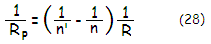with n and n' being the index of incidence and reflection/refraction, respectively, and R the optical surface radius of curvature (FIG. 60). It is called Petzval radius of curvature, or Petzval surface, in honor of Joseph Petzval, Hungarian mathematics professor from the 19th century who was the first to analyze it.

For mirror surface oriented to the left, n=1, n'=-1 and RP=R/2; this means that Petzval surface for a concave mirror is concentric with its surface, with the radius numerically equal to its focal length. In a multi-surface optical system, the curvatures induced by each surface combine into a final system's Petzval surface RPS, that can be either curved or flat. The curvatures generated by each surface simply add up as: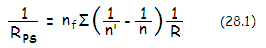nf being the final index of reflection/refraction at the last surface. For instance, a bi-convex lens with R1=1000mm, R2=-1000mm and n=1.5, thus n1=1, n1'=1.5 and n2=1.5, n2'=1 for the front and rear surface, respectively (for light traveling from left to right), would have Petzval radius of curvature RP=-1500mm. As the two relations imply, Petzval radius is independent of object and image distance.

For a single lens, Eq. 28.1 can be simplified to:

RP = -nf       (28.2)

where n is the glass refractive and f the lens focal length.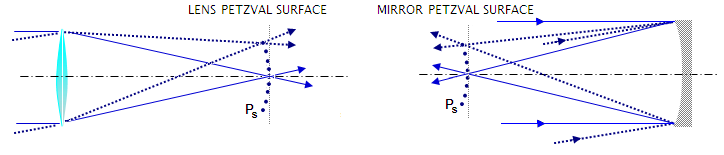FIGURE 60: Illustration of the Petzval field surface (Ps) in lens objective (left) and a concave mirror (right). Actual objectives usually do suffer from some astigmatism, resulting in altered best image surface. Note that the two curvatures, although of the same sign, are oriented differently with respect to the eyepiece. Even with the identical Petzval and astigmatism in both, they would still have field curvatures of different properties. In other words, the eyepiece optimized for one system, wouldn't be optimized for the other one.

For a doublet objective, Petzval radius of curvature is given by:

1/RP = (1/RP1)+(1/RP2) = (RP1+RP2)/RP1RP2         (29)

with 1 and 2 marking the front and rear lens, respectively. With astigmatism corrected it is the actual field curvature. The presence of astigmatism in a doublet will make the best image surface more strongly curved than the Petzval. This best - or median - image surface is formed by best astigmatic foci, midway between the astigmatic line foci. As mentioned, if the objective is an aplanat (w/corrected spherical aberration and coma), its astigmatism is not influenced by the position of aperture stop, and so is not its actual field curvature. Rough but useful approximation is that best image curvature of a typical contact doublet objective is ~1/3 of the focal length.

Petzval curvature for a pair of mirrors is given by: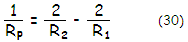with R1/2 being the surface radius of curvature for the first and second mirror, respectively. Such a pair is likely to have a fair amount of astigmatism, resulting in a significantly stronger best (median) image surface. This holds true for a single concave mirror as well. However, while change in the aperture stop position affects two-mirror systems little in regard to astigmatism, it is entirely different story with a single mirror. It is already mentioned in 2.3. Astigmatism how it changes for a concave mirror with the aperture stop position. There are consequences of it for the actual field curvature as well.

For mirror conics of 0 and smaller, the stop position for zero astigmatism, and best field curvature coinciding with the Petzval, is given by σ=[1-|K|]/(K+1), with α being the stop separation in units of the mirror radius of curvature, and K the mirror conic. It gives σ=1 for a sphere (K=0), and σ=0.5 for a paraboloid (K=-1, which makes the relation formally undefined, but the value of σ is found for K approaching -1). The field is astigmatic but the best surface is flat for the stop position σ=[1-0.5(1-K)]/(1+K), obtained by setting the right site of Eq. 39 to zero. It is also undefined for K=-1 (paraboloid), but as K approaches -1, σ approaches 0.25. Evidently, the field can't be formally flattened for K>1, or K<-1. For these conic ranges, median field curvature is only minimized with this stop position (for all practical purposes, it is flat for K of about -1.5 and greater, as well as for  K~1.1 and smaller).

Finally, best image surface curvature for the stop at the focal plane is given by Rm=R/(1-K), R being the mirror radius of curvature. As mentioned, for K<0 this is also the stop position cancelling astigmatism, so the best surface coincides with the Petzval surface.

As long as the image is observed on the Petzval surface, there is no optical path difference between the chief ray and the other rays, and field curvature doesn't cause image degradation. The wavefront is still spherical, thus the effect is merely displacing the point image from the focal plane. The displacement very seldom becomes as large as to affect visual observing by exceeding accommodation power of the eye. But it becomes a source of image degradation with flat detectors in imaging, when the longitudinal point image displacement results in defocus.

From Eq.  24, peak aberration coefficient (the P-V error) for defocus is given by P=Ld/8F2. In the case of field curvature, the longitudinal aberration Ld closely approximates the depth z of the image surface curvature. For the Petzval surface of the radius RP at the height h in the image plane, it is given by z=h2/2RP. Thus, the P-V wavefront error of defocus caused by field curvature with respect to the focal plane is given by: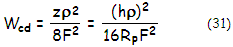with ρ being, as before, the ray height in the pupil for pupil radius normalized to 1. Note that defocus error caused by field curvature normally doesn't affect visual observing, due to eye accommodation. In the presence of astigmatism, point-images on best (median) image curvature combine the above defocus error with the error of astigmatism; in effect, defocus error is measured from best astigmatic focus to the image plane.

As mentioned, the presence of astigmatism significantly alters the image field curvature properties. The Petzval surface becomes fictitious, and the actual best image surface becomes the one containing best astigmatic foci. Due to the longitudinal extension of astigmatism, this image is split into sagittal, tangential and best, or median image surface sandwiched in between the first two. Relation between the Petzval on one side, and sagittal/tangential/median field curvature on the other is constant, as given by: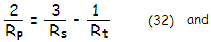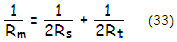with RP' Rs, Rt and Rm being the Petzval, sagittal, tangential and median field curvature, respectively. Sagittal surface is always between the Petzval and tangential, with the tangential surface being three times farther away from the Petzval than sagittal (FIG. 81).FIGURE 61: Illustration of image field curvatures of a concave mirror with the stop at the surface: tangential (T), median (M), sagittal (S) and Petzval (P) image surface are in a constant relationship with respect to the image plane, and to each other. Best, or median image surface contains best astigmatic foci (i.e. circles of least confusion). For a concave mirror with the stop at the surface, sagittal surface is flat, coinciding with the Gaussian (paraxial) image plane. Tangential (vertical) and sagittal planes are perpendicular to each other, and the plane in which rays focus at the median surface is midway between them. Both, longitudinal astigmatism and field curvature increase with the square of the field angle α. All image surfaces are paraboloidal.

This implies that a system with given longitudinal defocus due to its Petzval curvature will have flat median astigmatic surface when the longitudinal astigmatism equals longitudinal Petzval defocus. Field flattening by introduction of astigmatism is often used in optical systems. Since for given longitudinal aberration astigmatism produces half as large blur as defocus, with its RMS error smaller by a factor of 0.68, there is substantial gain in the quality over flat detectors.

For a singlet lens, sagittal and tangential image curvature, respectively, are given by: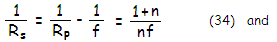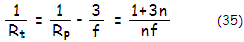f being the singlet's focal length and n the refractive index. Obviously, median curvature is (1/Rm)=(1+2n)/nf. Since astigmatism doesn't change with object distance, the image surface radii scale with the image distance (as mentioned, astigmatism in aplanatic doublets also doesn't change with the stop position).

For a doublet, the respective image curvatures are simply a sum for the two lenses:

1/RsD=[(1+n1)/n1f1]+[(1+n2)/n2f2],

1/RtD=[(1+3n1)/n1f1]+[(1+3n2)/n2f2],  and

1/RmD=[(1+2n1)/n1f1]+[(1+2n2)/n2f2].

Note that the focal length of a negative lens is numerically negative for incident light traveling left to right.

For a mirror of radius of curvature R with the stop at the surface, sagittal and tangential image curvatures, respectively, are given by: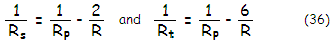which, with RP=R/2, can be written as 1/Rs=0 (implying Rs= ∞), and 1/Rt=-4/R. The median image surface, given by Eq. 33 as one half of the sum of sagittal and tangential curvatures, is 1/Rm=-2/R, equaling mirror focal length (as absolute value; the plus sign implies that it is concave toward mirror, for mirror oriented to the left). In other words, the median surface has the same curvature radius as the Petzval, but of opposite sign.

Similarly to the doublet objective, astigmatic curvatures for a pair of mirrors are given by a sum of the respective curvatures for each mirror.

With the aperture stop axially displaced from the mirror to a distance σ in units of the mirror radius of curvature, the astigmatic image curvatures become: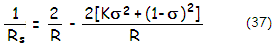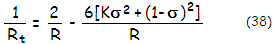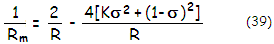with K being the mirror conic, and 2/R=1/RP.

For a pair of mirrors, the curvatures are combined: the sum of Petzval curvatures results in a system Petzval, which is then used to obtain sagittal and tangential curvatures. The former from

1/Rs=(1/RP)+2as/n'α2,

for the system astigmatism coefficient (primary oriented to the left), as=(α2/R1)+α2[(K2/R2)+(1-σ2)2]/R2, with α, K2, σ2, R2 and n' being the field angle, secondary mirror conic, relative stop separation, radius of curvature and index of reflection at the final surface, respectively. For the latter, from

1/Rt=(1/RP)+6as/n'α2.

For the secondary, the relative stop distance σ2 is the primary-to-secondary separation (since the primary acts as an aperture stop - or entrance pupil - for the secondary) in units of the primary mirror radius of curvature.

▐